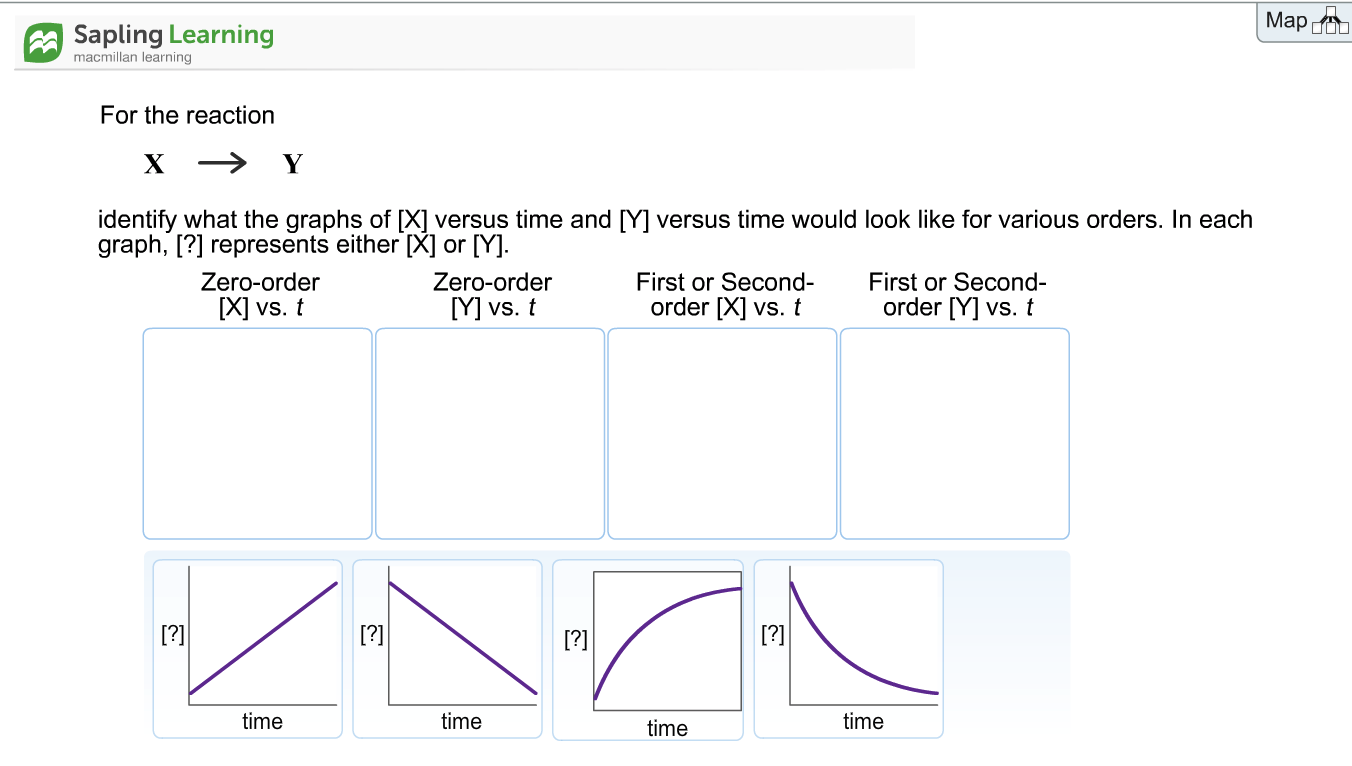MapSapling Learningmacmillan learningFor the reactionidentify what the graphs of [X] versus time and [M versus time would look like for various orders. In eachgraph, [?] represents either X or [Y.Zero-order[X] vs. tZero-order[M vs. tFirst or Second- First or Second-order [X] vs. t order [] vs.ttimetimetimetime

Questionhelp_outlineImage TranscriptioncloseMap Sapling Learning macmillan learning For the reaction identify what the graphs of [X] versus time and [M versus time would look like for various orders. In each graph, [?] represents either X or [Y. Zero-order [X] vs. t Zero-order [M vs. t First or Second- First or Second- order [X] vs. t order [] vs.t time time time time fullscreen
Step 1

In the given reaction,

X is a reactant and Y is a product.

First Graph:

Zero-order [Y] versus time is a straight line with positive slope.

Step 2

Second Graph:

Zero-order [X] versus time is a straight line with negative slope.

Step 3

Third Graph:

First-order/second-order [Y] versus time is an exponentially increasing ...

Want to see the full answer?

See Solution

Want to see this answer and more?

Our solutions are written by experts, many with advanced degrees, and available 24/7

See Solution
Tagged in

Chemistry